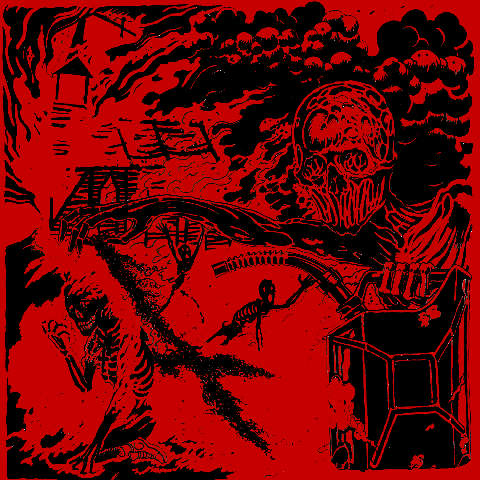## VM-UNDERGROUND

### Extreme Metal Fanzine est. 2012

Filter by: band
[%] - [[0-9]] - [A] - [B] - [C] - [D] - [E] - [F] - [G] - [H] - [I] - [J] - [K] - [L] - [M] - [N] - [O] - [P] - [Q] - [R] - [S] - [T] - [U] - [V] - [W] - [X] - [Y] - [Z]
Filter by: label
[[0-9]] - [A] - [B] - [C] - [D] - [E] - [F] - [G] - [H] - [I] - [J] - [K] - [L] - [M] - [N] - [O] - [P] - [Q] - [R] - [S] - [T] - [U] - [V] - [W] - [X] - [Y] - [Z]
Filter by: style
[B] - [C] - [D] - [E] - [F] - [G] - [H] - [I] - [P] - [S] - [T] - [V]
Filter by: country
[A] - [B] - [C] - [D] - [E] - [F] - [G] - [I] - [L] - [M] - [N] - [P] - [R] - [S] - [T] - [U]
Filter by: vmu-author
[A] - [B] - [C] - [D] - [E] - [F] - [G] - [H] - [I] - [J] - [K] - [L] - [M] - [N] - [O] - [P] - [R] - [S] - [T] - [V] - [X] - [Y] - [Z]

# Wan – Gammal är äldst [EP]## Info

Wan are here to create chaos! “Gammal Är Äldst” is the strong bear of the North, primitive disturbing and harsh sound as it should be. High pick distortion on guitars and aggressive harsh vocals straight from the abyssic old noisy sound. Drums are bitty and fast in a more punk attitude recalling early Venom, Bathory and some Von influences from their depths. If you are ready to get some real old school feeling test yourselves with this. But Beware this release is NOT for gentle ears. This is for true listeners of the primitive dark arts!! (P.)